# Built-up steel section properties using some good old Python (Part 3)

In this part we cover slapping some plates on the sides of an I-Section before unleashing the welder to make some fire.

But first I wanted to touch on an important aspect, in the previous post we discussed the strengthening elements being in intimate contact and that fact that this could lead to incorrect results.

The other aspect I wanted to discuss regarding the correctness of calculated torsional properties is whether or not you have continuous or intermittent welding. Why does this matter?

Because it does all right … let me explain, if you have continuous welding the result we came up with in the previous post is correct with respect to the torsion constant, we have some fundamental behaviour where the plates can act integrally with each other to transfer the shear flow around the perimeter of the combined section.

But as soon as you have intermittent welds the shear flow around the perimeter of the section acts quite differently, having to stay within the individual elements because of the lack of full interconnection. If you cut a section where there are no welds you essentially have the strengthening element and the original section with no interconnection. So obviously shear flow cannot transfer between two things that are disconnected unless you’re able to warp the laws of physics.

So, what does this mean for our calculated section properties. If you have intermittent welding, the torsion constant for a combined section is simply the sum of all the constituent bits. For example: –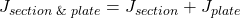Don’t forget this, it’s relatively important and often overlooked with all the commercial offerings where you can put multiple ‘things’ together and get it to report anything based on the overarching assumption that anything you put together is in full and intimate contact over the entire cross-section & member length.

We know better than those guys.

### The code

Back to the subject of this post, let’s not keep the welder waiting!…..

This approach of slapping some plates on either side of the flanges effectively turns your I-Section into a closed section with an internal web. Obviously greatly increasing the torsional properties in the process (if you’re using continuous welding).

But on the balance of things not an extremely efficient a way of increasing major axis bending capacity as putting metal at the extremities on top and bottom flanges. But for axial strengthening of a member, it’s usually another matter altogether. For axial buckling it’s probably the most effective (and common) way of increasing the buckling capacity of a column about its minor axis.

import copy
import sectionproperties.pre.sections as sections
from sectionproperties.analysis.cross_section import CrossSection

# -------------------------------------------------------------
# Inputs
# -------------------------------------------------------------

d = 308  # I section depth
b_f = 305  # I-section flange width
t_f = 15.4  # I-section flange thickness
t_w = 9.9  # I-section web thickness
r_1 = 16.5  # I-section root radii

d_p = 330  # strengthening plate depth
b_p = 16  # strengthening plate thickness

gap = 0.5  # gap between plate and section

weld_size = 8  # weld size

n_r = 50  # number of points considered around root radii

mesh_area = 10  # max mesh size

# -------------------------------------------------------------
# Calculations
# -------------------------------------------------------------
# create constituent geometry
weld = sections.CustomSection(
points=[[0, 0], [weld_size, 0], [0, weld_size]], facets=[[0, 1], [1, 2], [2, 0]], holes=[], control_points=[[weld_size / 3, weld_size / 3]]
)

if d_p < d and not gap == 0:
weld_horiz_move = 0
else:
weld_horiz_move = gap

# I-Section beam
geometry1 = sections.ISection(d=d, b=b_f, t_f=t_f, t_w=t_w, r=r_1, n_r=n_r, shift=[-b_f / 2, -d / 2])

# strengthening plates 1 & 2
geometry2 = sections.RectangularSection(d=d_p, b=b_p, shift=[-b_f / 2 - b_p - gap, -d_p / 2])
geometry3 = sections.RectangularSection(d=d_p, b=b_p, shift=[b_f / 2 + gap, -d_p / 2])

# weld 1
geometry4 = copy.deepcopy(weld)
geometry4.shift = [-b_f / 2 - weld_horiz_move, min(d, d_p) / 2]
geometry4.shift_section()

# weld 2
geometry5 = copy.deepcopy(geometry4)
geometry5.mirror_section(axis='x', mirror_point=[0, 0])

# weld 3
geometry6 = copy.deepcopy(geometry4)
geometry6.mirror_section(axis='y', mirror_point=[0, 0])

# weld 4
geometry7 = copy.deepcopy(geometry5)
geometry7.mirror_section(axis='y', mirror_point=[0, 0])

# flip welds if plates are shorter than section depth
if d_p < d:
x = b_f / 2
geometry4.mirror_section(axis='y', mirror_point=[-x, 0])
geometry5.mirror_section(axis='y', mirror_point=[-x, 0])
geometry6.mirror_section(axis='y', mirror_point=[x, 0])
geometry7.mirror_section(axis='y', mirror_point=[x, 0])

# total number of individual geometry elements
geo_number = 7

# assemble geometry list
geo_list = [globals()[f'geometry{i}'] for i in range(1, geo_number + 1)]

# create merged section
geometry = sections.MergedSection(geo_list)

# clean geometry
geometry.clean_geometry(verbose=True)

# create mesh
mesh = geometry.create_mesh(mesh_sizes=[mesh_area] * geo_number)

# create section
section = CrossSection(geometry, mesh)

# calculate results
section.calculate_geometric_properties()
section.calculate_plastic_properties()
section.calculate_warping_properties()

# plot results
section.plot_mesh()
section.plot_centroids()

# display all results
section.display_results(fmt='.3f')


The code will do 2 flavours of strengthening depending on the depth of the plates vs section depth, as illustrated below: –

### Some thoughts…

Given we’ve effectively created a closed section…. it’s also interesting to determine if we can simplify the derivation of the torsion properties of our new closed section down to assessing an equivalent rectangular tube for calculating torsion properties, since we more or less created one if we ditch/ignore the web of the I-Section.

Standard formulas exist for this of course, but do we lose or gain anything by considering the actual configuration versus a closed tube.

Well let’s see by comparing a closed tube, and the above member. Then we’ll calculate it by hand using the following random formula I found on the internet and see if my fear of random internet formulas is well founded (or not): –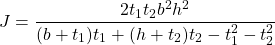Where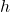and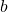is the distance between the centreline of the walls of our equivalent tube, and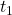and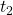are the respective thicknesses of these walls.

Let’s check the first example above with a 310UC97 with 2 x 330 x 16PL’s extending past the flanges: –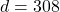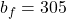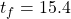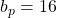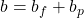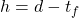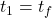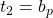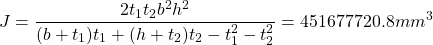From our section-properties analysis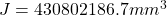with a 5mm mesh and 50 points around the curves, that’s good enough I guess for a hand check, being about 4% higher by the internet formula in this case. Your milage may vary depending on an individual configuration.

If you remove the small gap between the section and remove the welds, you’ll get a larger value from the analysis vs the internet formula. Still within a few percent, totally dependent on assumptions at the end of the day.

So, if you’re after the torsion constant, at a pinch you now have a good rough estimate for it from a random internet formula. Who needs all this fancy python code after all ….

You might be asking in this case what occurs with the warping constant, who cares, for design it is typically taken as zero for closed sections (it isn’t actually zero, but relative to St. Venants torsion, warping is many times more insignificant when considering Lateral Torsional Buckling (LTB) for closed sections).

Stay frosty until part 4